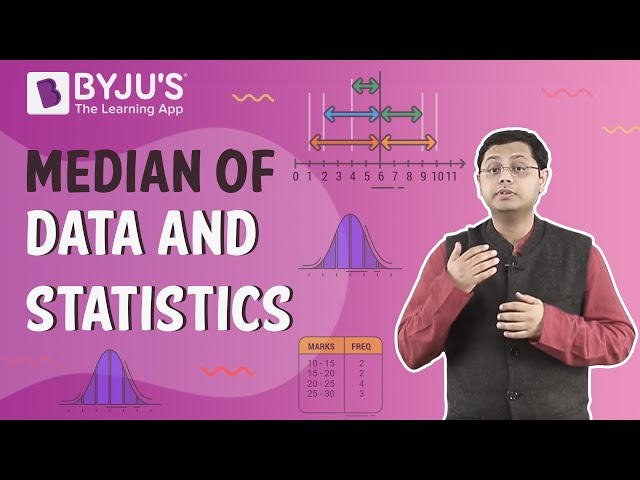# Difference Between Mean Median and Mode

The difference between mean, median and mode are:

• Mean is the average value of the given observations
• Median is the middle value of the given observations
• Mode is the most repeated value in the given observation

## What is Mean, Median, and Mode?

The mean is the average where the sum of all the numbers is divided by the total number of numbers, whereas the median is the middle value in the list of given numbers numerically ordered from smallest to biggest and mode is the value of the number which occurs most often in the list. You can learn more about it here:

## What are the differences between Mean, Median, and Mode?

These three terms are related to each other. There’s a relationship between mean, median and mode and is called an empirical relationship between them. Below are some of the most integral differences between the mean, median and mode.

 Sl. No. Mean Median Mode 1. The average taken for a set of numbers is called a mean. The middle value in the data set is called the Median. The number that occurs the most in a given list of numbers is called a mode. 2. Add all of the numbers together and divide the sum by the total number of values. Place all the given numbers in an ascending order It shows the frequency of occurrence. 3. The result is the mean or average score. The next step is to find the middle number on the list. It is called the median. We can have more than one mode or no mode at all. 4. Example: To find the average of the four numbers 2, 4, 6, and 8, we need to add the number first. 2 + 4 + 6+ 8 = 20 Divide the sum by the total number of numbers, i. e 4. 20/4 = 5 is the average or mean Example: 4, 2, 8, 10, 19. Arrange the numbers in ascending order. i .e., 2, 4, 8, 10, 19. As the total numbers are 5, so the middle number 8 is the median here. Example: 3, 3, 5, 6, 7, 7, 8, 1, 1, 1, 4, 5, 6. Find the frequency of each number. For number 3, it’s 2. For 5, it’s 2. For 6, it’s 2. For 7, it’s 2. For 8, it’s one. For 1, it’s 3. For 4, it’s 1. The number with the highest frequency is the mode. Hence, the mode of the given sequence of numbers is 1.

## Video Lesson on Median of DataRegister at BYJU’S to learn about other mathematical concepts in a fun and engaging way.

## Frequently Asked Questions on the Difference Between Mean, Median and Mode

Q1

### What are the three measures of central tendencies?

The three measures of central tendencies are mean, median and mode.
Q2

### What is mean in statistics? Give an example.

The mean is the average of given data values. The formula to calculate the mean value is:
Mean = Sum of observation/Number of observations
For example, Mean of 2, 5, 6, 7, 8 is, (2+5+6+7+8)/5 = 28/5 = 5.6
Q3

### What is a median? Explain with an example.

The median is the middle value of a given observation. For example, 23, 33, 43, 63, and 53 is a set of observations; then, to find the median, we need to arrange the given values in an order (ascending or descending). Hence, we get
23, 33, 43, 53, 63
Therefore, Median = 43
Q4

### What is a mode? Give an example.

Mode represents the value which is repeated the maximum number of times in a given set of observations. For example, 11, 12, 13, 13, 14, and 15 are the set of data. Here, the number 13 is repeated twice and is considered to be the mode value.
Q5

### What are the mean, median and mode formulas?

Mean = Sum of observation/Number of observation
Median = {(n+1)/2}th term when n is odd
& Median = [(n/2)th term + {(n/2)+1}th]/2 when n is even
Mode = Value repeated the maximum number of times.
Quiz on Difference Between Mean Median and Mode